# PSEB 3rd Class Maths Solutions Chapter 9 Time

Punjab State Board PSEB 3rd Class Maths Book Solutions Chapter 9 Time Textbook Exercise Questions and Answers.

## PSEB Solutions for Class 3 Maths Chapter 9 Time

PAGE 179:

Question 1.
When does the sun rise?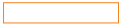Solution.
In the morning

Question 2.
When do we come back home from school?Solution.
In the afternoon.

Question 3.
In which month summer vacations start?Solution.
In June

Question 4.
How many days are there in a week?Solution.
7 days.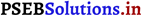PAGE 181:
Let’s do: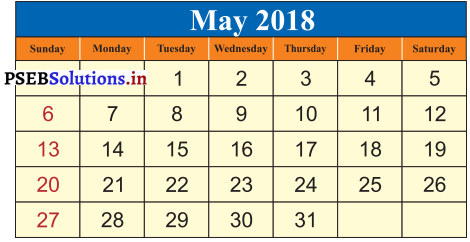Question 1.
How many days are there in the month of May ?
(a 28
(b) 29
(c) 30
(d) 31
Solution.
31

Question 2.
Which day falls on 5th May ?
(a) Monday
(b) Tuesday
(c) Sunday
(d) Friday
Solution.
(c) Sunday

Question 3.
Which day falls on 28th May?
(a) Sunday
(b) Tuesday
(c) Thursday
(d) Saturday
Solution.
(b) TuesdayQuestion 4.
What date is oil the first Sunday of the month of May?
(a) 1
(b) 2
(c) 5
(d) 6
Solution.
(c) 5

Question 5.
What date is it on the first day in May?
(a) 1
(b) 2
(c) 3
(d) 6
Solution.
(d) 6

Question 6.
Which day is on 31 May?
(a) Sunday
(b) Monday
(c) Thursday
(d) Friday
Solution.
(d) FridayPAGE 182: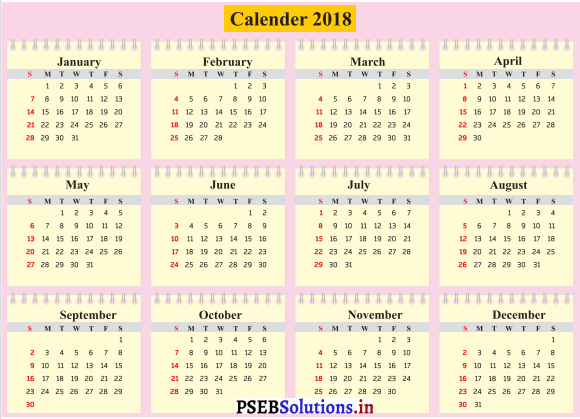Question 1.
See calender and Fill in the date in these blanks: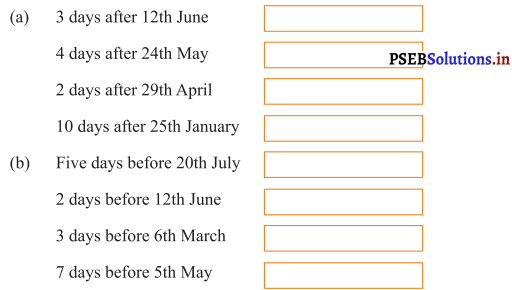Solution.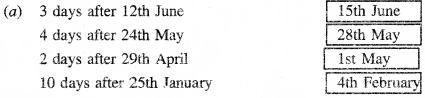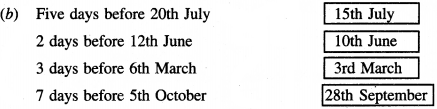PAGE 186:

Question 2.
Draw the hour and minute hand to show the given time:

(1) How many Sundays are there in the month of October?
Solution.
4

(2) What day of week is on 6th September?
Solution.
Friday

(3) What day of week is on 25th October?
Solution.
Friday

(4) In which month you were born?
Solution.
Write yourself

(5) What day of the week is 18th May?
Solution.
Saturday(6) How many Sundays are there in the month of May?
Solution.
4

(7) How many Thursdays are there in the month of August?
Solution.
5

(8) What day of the week is on 1st May?
Solution.
Wednesday

(9) What is the last day of May?
Solution.
Friday

(10) How many days are in the month of February?
Solution.
28PAGE 184:
Let’s do:

(1) What does the Long hand on the clock tells us?
Solution.

(2) What does the smallest hand of the clock tells us?
Solution.

(3) What does the hand which moves fast tells us?
Solution.PAGE 184:
Let’s do:

Question 1.
Write the time shown on each clock: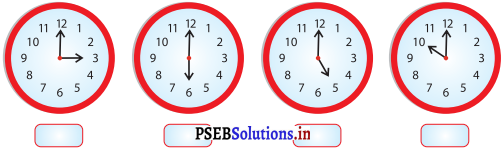Solution.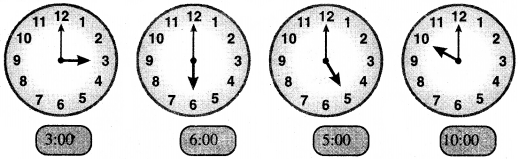PAGE 186: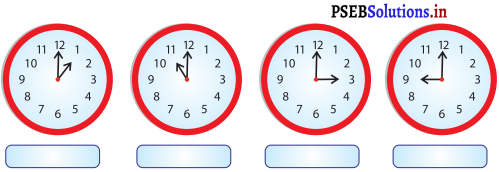Solution.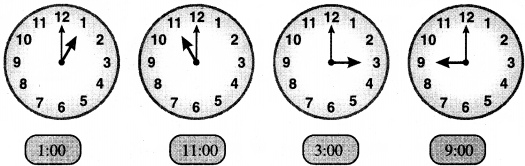Question 2.
Draw the hour and minute hand to show the given time: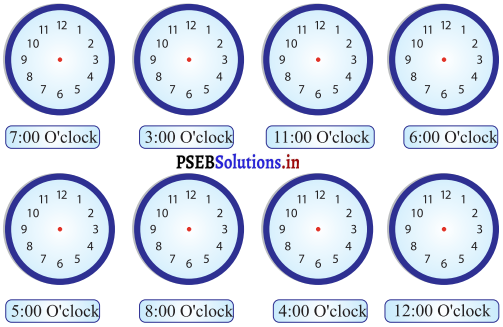Solution.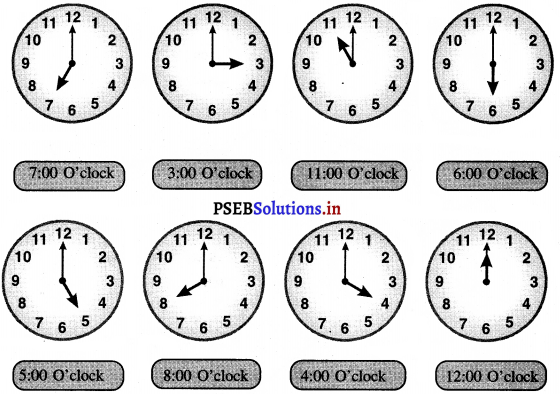Question 3.
Read the time shown in the central clock and write the correct time on the left and the right hand clocks:

i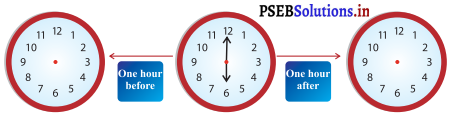Solution.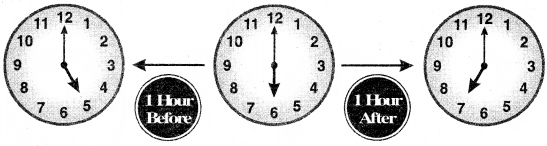Question 4.
Match the columns with time shown on the clock: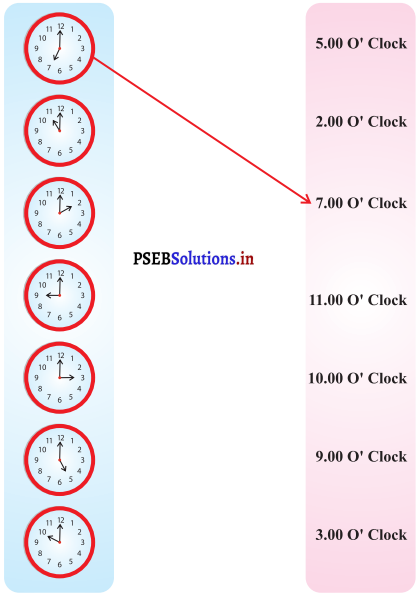Solution.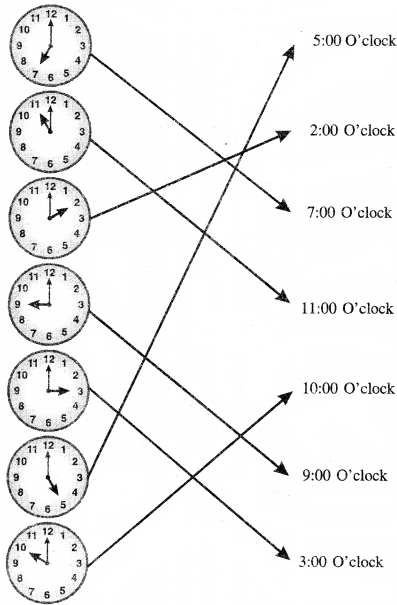Worksheet:

Question 1.
Fill in the blanks:
(a) There are _______ days in an odinary year.
Solution.
365

(b) There are _______ months in a year.
Solution.
12

(c) There are _______ days in a week.
Solution.
7Question 2.
(a) How many days are there in the month of April?
Solution.
30 Days.

(b) How many days are there in the month of May?
Solution.
31 Days.

(c) In which month you were born?
Solution.
Write your own month of birth.Question 3.
Write the time shown on each clock :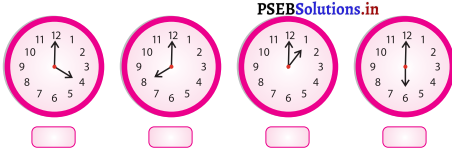Solution.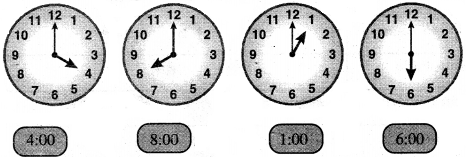Question 4.
Draw the hour and the minute hand in the clock as per the time written under each clock: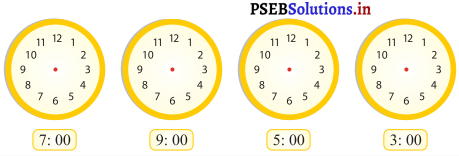Solution.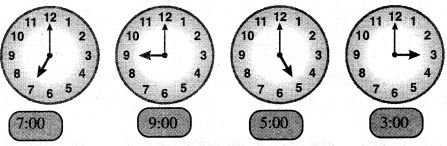Multiple Choice Questions:

Question 1.
Which of the following is not unit of time?
(a) Second
(b) Hour
(c) Minute
(d) Gram
Solution.
(d) Gram.

Question 2.
The number of days in a leap year is:
(a) 364
(b) 365
(c) 366
(d) 367
Solution.
(c) 366.